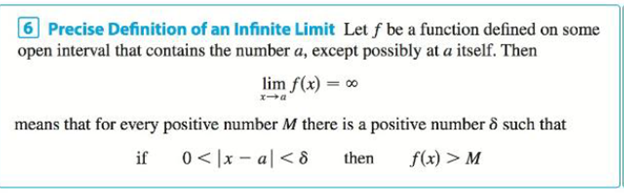Chapter 2.4, Problem 42E### Single Variable Calculus: Early Tr...

8th Edition
James Stewart
ISBN: 9781305270343

#### Solutions

Chapter
Section### Single Variable Calculus: Early Tr...

8th Edition
James Stewart
ISBN: 9781305270343
Textbook Problem

# Prove, using Definition 6, that lim x → − 3 1 ( x + 3 ) 4 = ∞ Definition 6To determine

To prove: The limit of a function limx3(1(x+3)4) is equal to by using the definition of a infinite limit.

Explanation

Definition used:

“Let f be a function defined on some open interval that contains the number a, except possibly at a itself. Then limxaf(x)= means that for every positive number M there is a positive number δ such that if 0<|xa|<δ then f(x)>M”.

To guess: The number δ.

Let ε be a given positive integer. Here, a=3 and f(x)=1(x+3)4.

By the definition of infinite limit it is enough to find a number δ such that if 0<|x(3)|<δ then 1(x+3)4>M

Consider 1(x+3)4>M

1(x+3)4>M1(x(3))4>M(x(3))4<1M|x(3)|4<1M

Take fourth root on both sides of the inequality,

|x(3)|44<1M4|x(3)|<1M4

Thus, find a number δ such that 0<|x(3)|<δ then |x(3)|<1M4

### Still sussing out bartleby?

Check out a sample textbook solution.

See a sample solution

#### The Solution to Your Study Problems

Bartleby provides explanations to thousands of textbook problems written by our experts, many with advanced degrees!

Get Started

#### In Exercises 13-20, sketch a set of coordinate axes and plot each point. 20. (1.2, 3.4)

Applied Calculus for the Managerial, Life, and Social Sciences: A Brief Approach

#### In problems 23-58, perform the indicated operations and simplify. 40.

Mathematical Applications for the Management, Life, and Social Sciences

#### For

Study Guide for Stewart's Multivariable Calculus, 8th